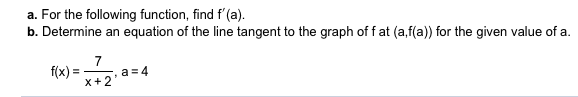# a. For the following function, find f'(a)b. Determine an equation of the line tangent to the graph of f at (a,f(a) for the given value of a7a 4f(x)x+2

Question
6 viewshelp_outlineImage Transcriptionclosea. For the following function, find f'(a) b. Determine an equation of the line tangent to the graph of f at (a,f(a) for the given value of a 7 a 4 f(x) x+2 fullscreen
check_circle

Step 1

Consider the given function,

Step 2

a.

Differentiate the given function,

Step 3

To find f’(a) , where a=4

Substitute ...

### Want to see the full answer?

See Solution

#### Want to see this answer and more?

Solutions are written by subject experts who are available 24/7. Questions are typically answered within 1 hour.*

See Solution
*Response times may vary by subject and question.
Tagged in

### Calculus